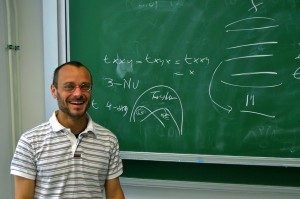# Simone Bova

## Expression Complexity of Conjunctive Queries

VCLA will host a talk by Simone Bova (Vanderbilt University) on Friday, 23rd March 2012.

 DATE: Friday, March 23, 2012 TIME: 12:30 VENUE: Seminar room von NeumannABSTRACT

A fundamental algorithmic problem from logic, with a variety of applications in computer science, is the model checking problem: Given a second-order sentence (on a purely relational signature) and a finite relational structure, decide whether the sentence is true in the structure. If both the sentence and the graph are given in input, the model checking problem is computationally hard (its combined complexity is PSPACE-complete), which raises the question -- which restrictions, imposed over input sentences or structures, warrant computational tractability? For instance, if a sentence in the language of graphs is fixed as a parameter, then the (data) complexity of the model checking problem is tractable.

An important restricted version of the model checking problem is the constraint satisfaction problem (CSP): a finite relational structure is fixed as a parameter, and the input sentence is first-order primitive positive (primitive positive formulas are also known as conjunctive queries in database theory). In the Nineties, Jeavons and coauthors discover that the (expression) complexity of the CSP over a fixed structure B is completely determined by a certain finite algebra naturally corresponding to B, and conjecture that the CSP on B is tractable if this algebra is nontrivial (in a sense that can be made precise), and NP-complete otherwise.

In this talk, we discuss the algebraic approach to the complexity of the CSP, emphasizing its applicability to the design of efficient algorithms for the CSP, and to the complexity investigation of several variants of the CSP (quantified CSP, soft CSP, and others).